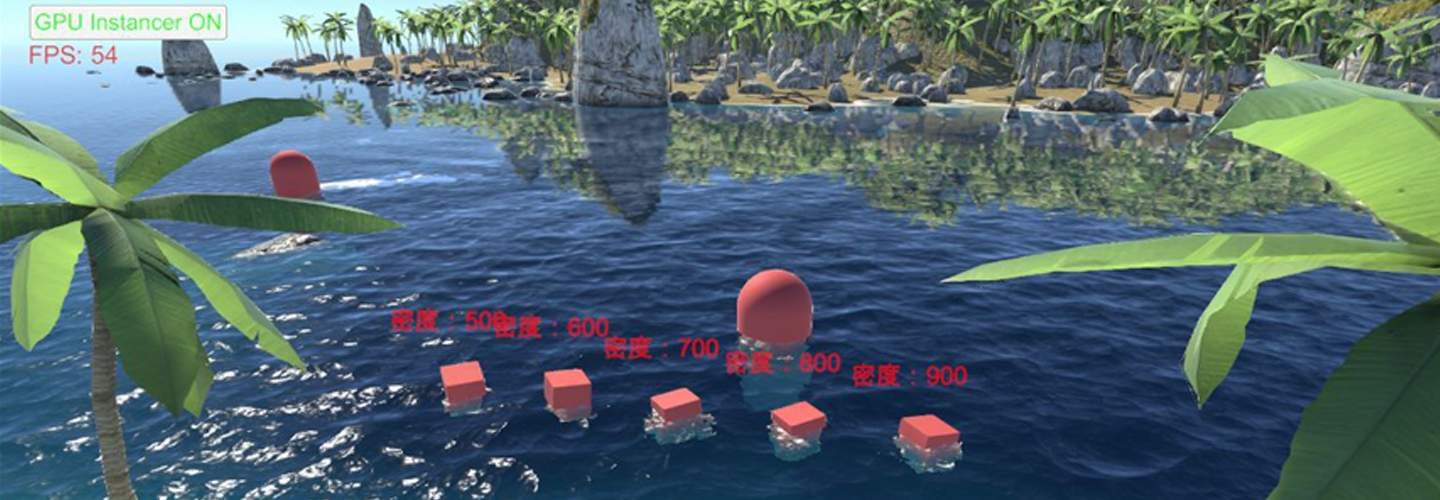# 水体渲染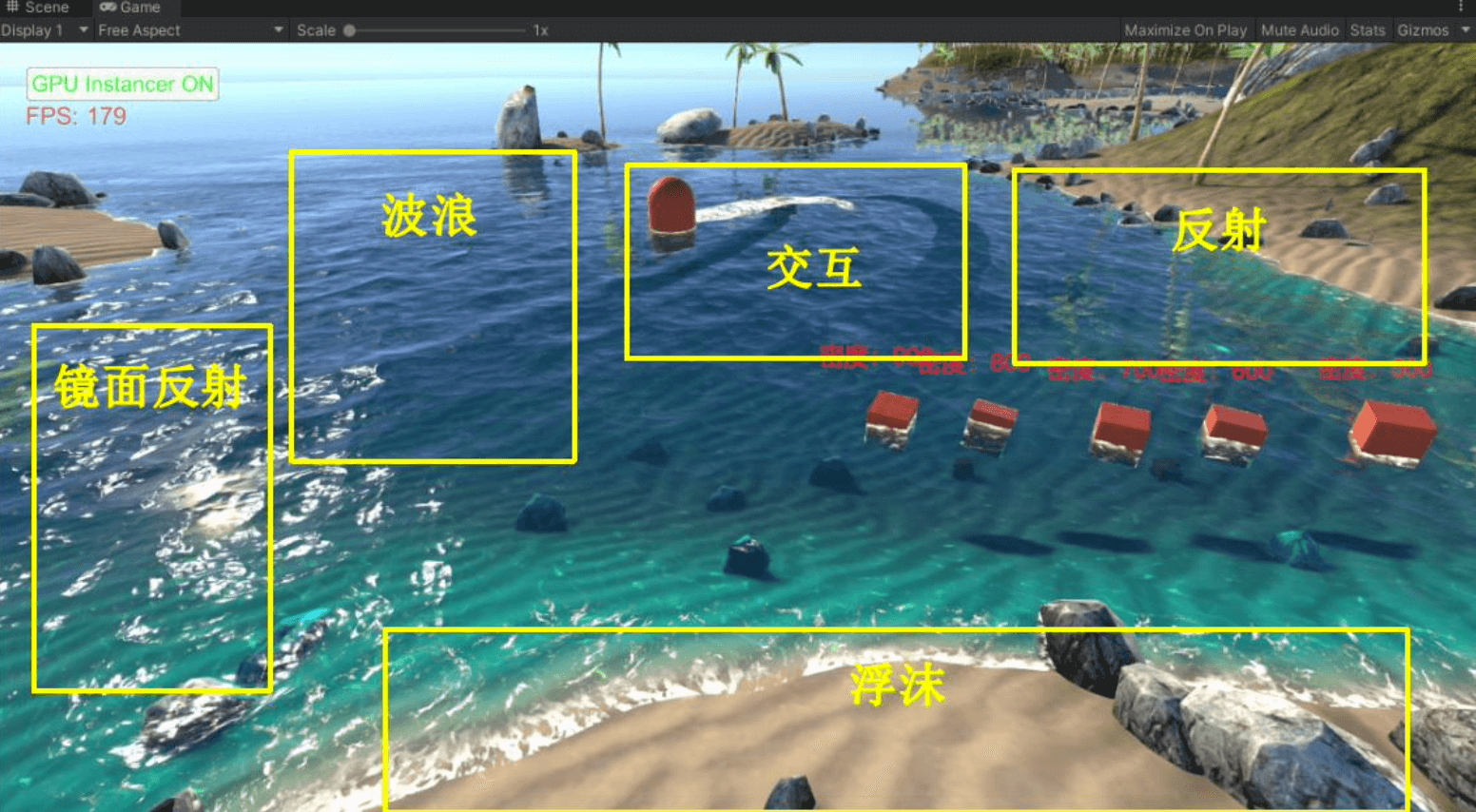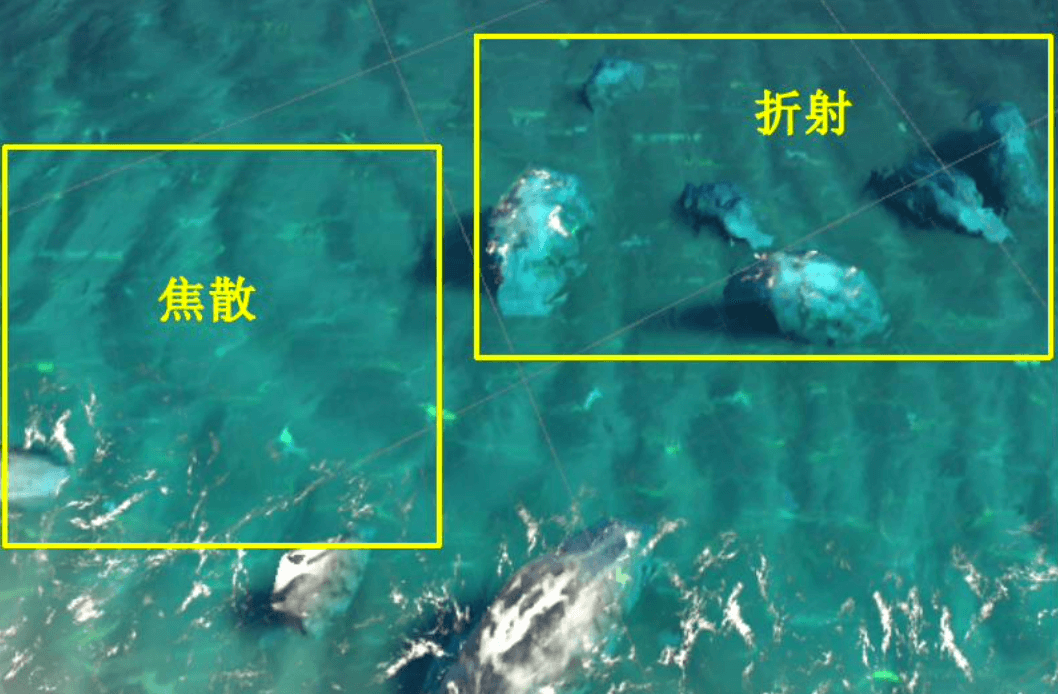### 一、波浪

1. LOD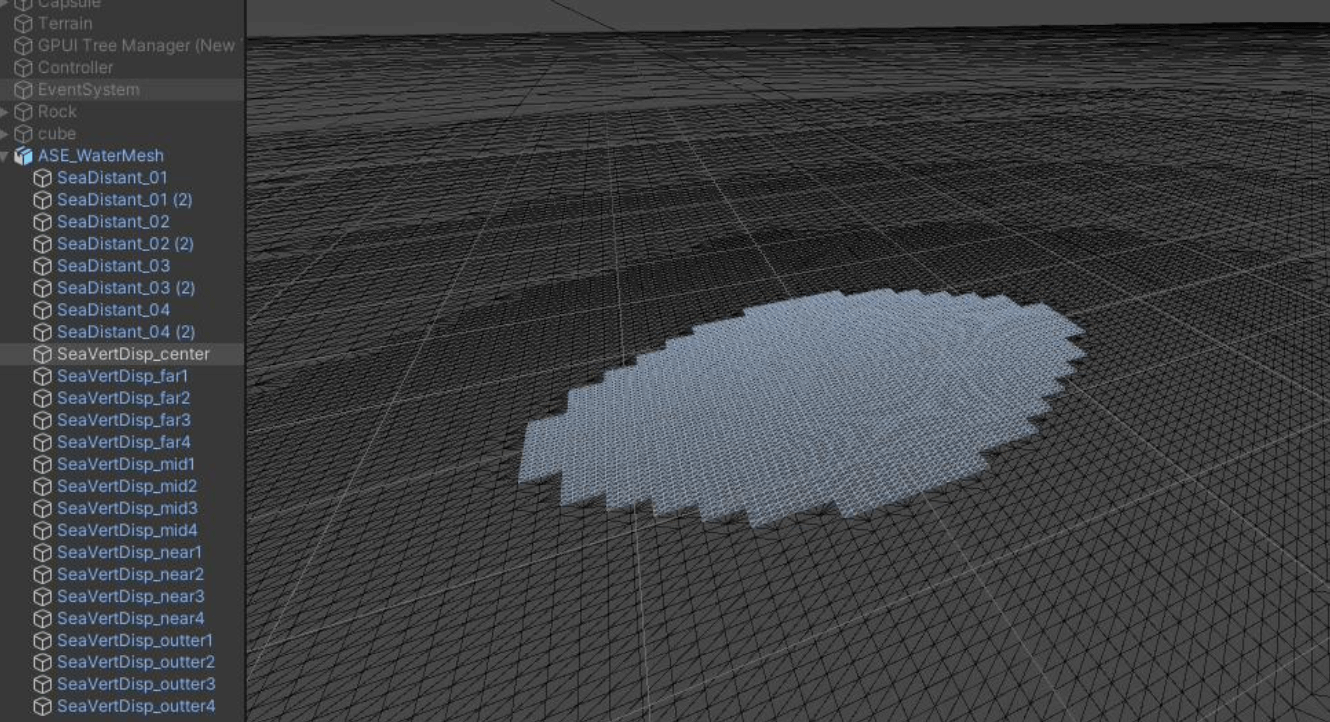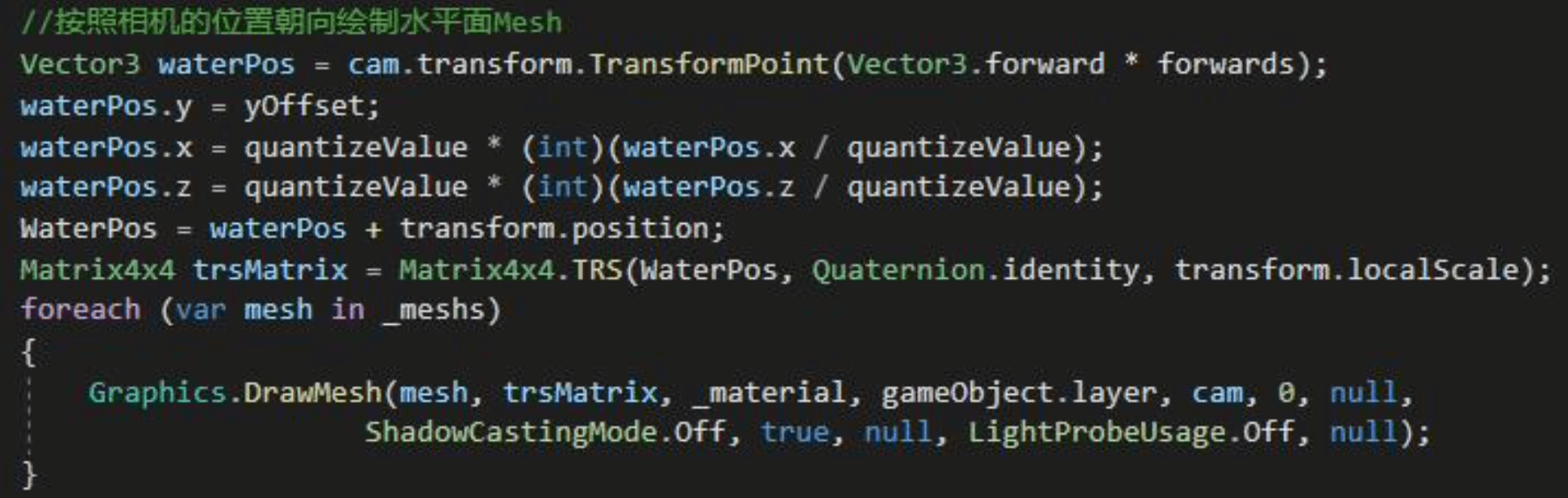2. 顶点动画

2.1 单个Sin函数

Wi(x,y,t) = Aisin(Di(x,y)wi+tΦi)

Wi：修改后的顶点位置。输入为x,y坐标（水面顶点的x,z）以及时间t，输出为变换后的z坐标（水面顶点的y）
Ai：控制水的振幅
Di：波浪移动的方向，与(x,y)点乘得到某一方向上的分量
wi：频率，和波长互为倒数，控制水的波长
Φi：与t相乘代表波峰的移动距离，控制水的移动速度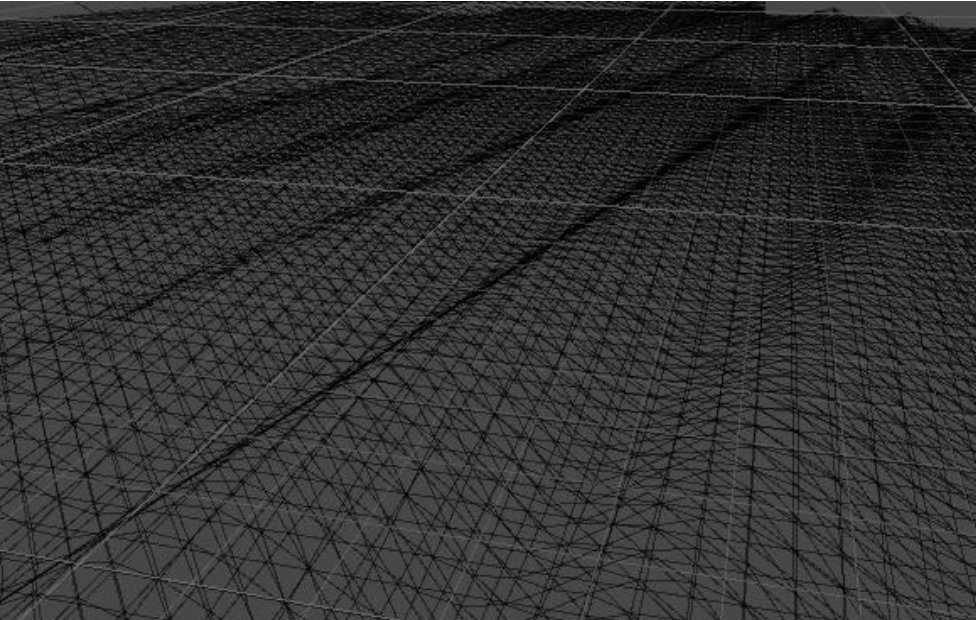P(x,y,t) = (x,y,Wi(x,y,t)) ，其中Wi(x,y,t)即刚才的顶点高度。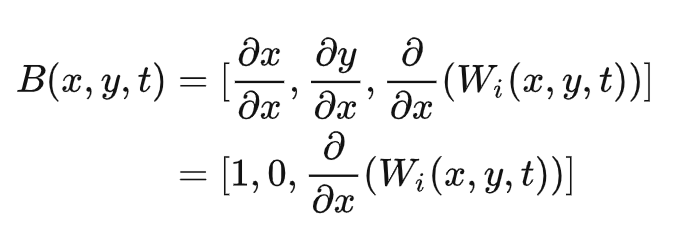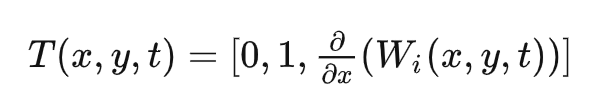B*T得到N：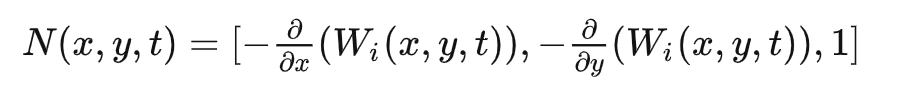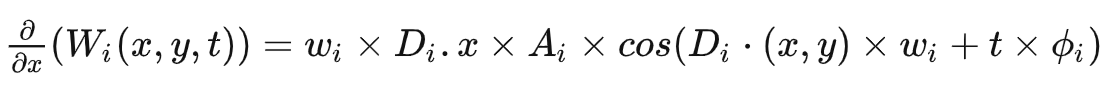``````//单个Sin波浪
WaveStruct SinWave(half2 pos,float waveCountMulti, half amplitude, half angle, half wavelength)
{
WaveStruct waveOut;
float time = _Time.y;
half w = 6.28318 / wavelength;
half wSpeed = sqrt(9.8 * w);
half2 direction = half2(sin(angle), cos(angle));
half dir = dot(direction, pos);
half calc = dir * w + time * wSpeed; // the wave calculation
half cosCalc = cos(calc);
half sinCalc = sin(calc);
waveOut.position = 0;
waveOut.position.y = amplitude * sinCalc*waveCountMulti;
waveOut.normal = normalize(float3(
-w*direction.x*amplitude*sinCalc,
1,
-w*direction.y*amplitude*sinCalc
)) * waveCountMulti;
return waveOut;
}
``````

2.2 叠加Sin函数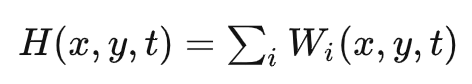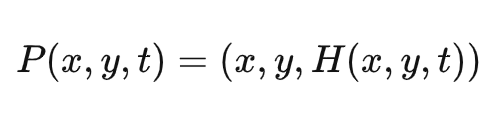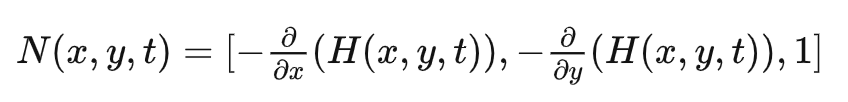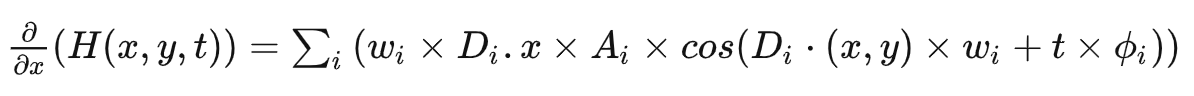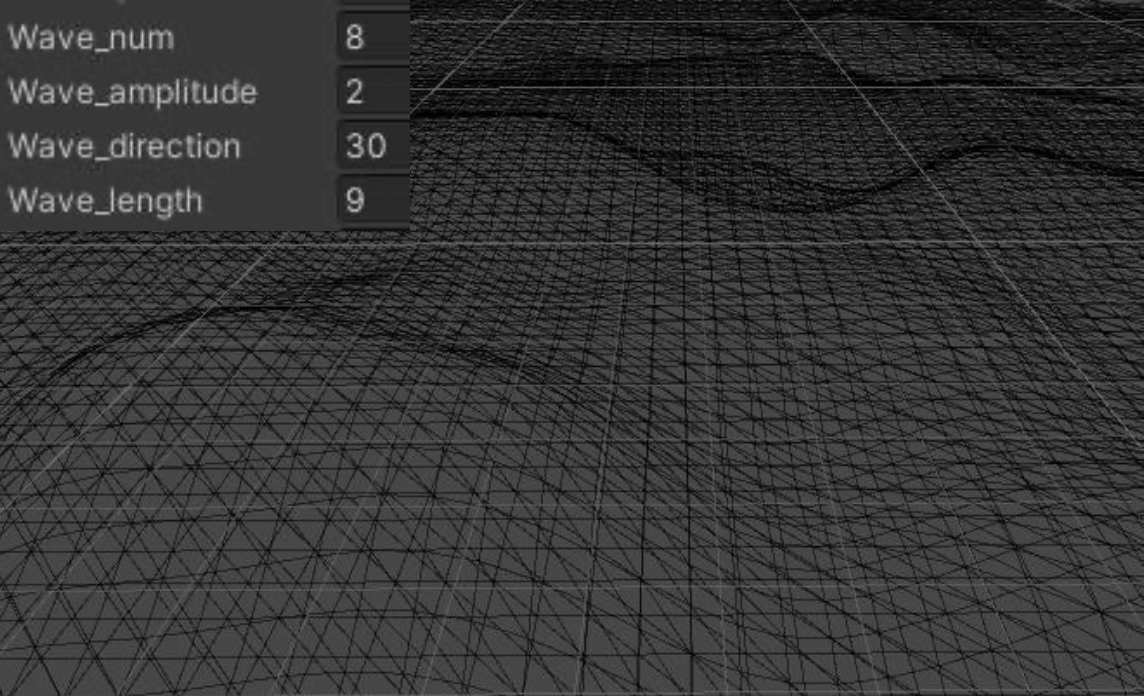2.3 Gerstner叠加波浪
Sin函数的变种，可以使得波谷更加平坦，波峰更陡峭，更好地模拟波浪。
Sin函数中的P(x,y,t)只会修改其z值，而Gerstner中还需要将x,y坐标向波峰位置移动。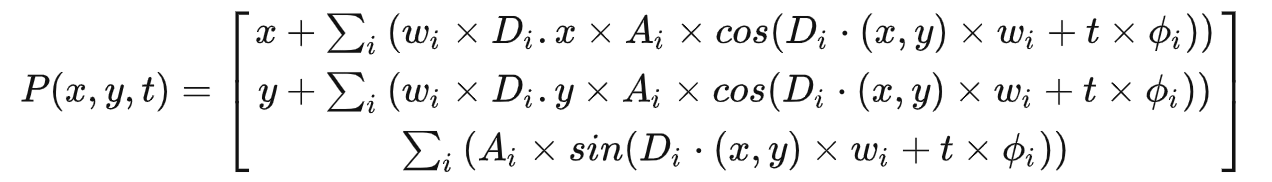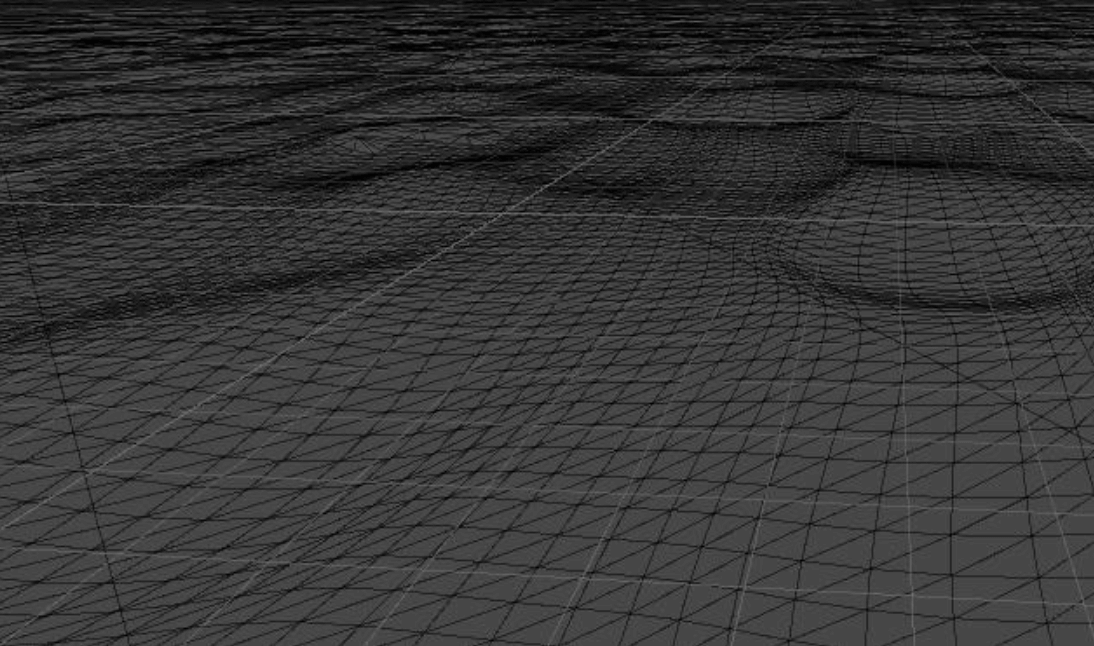### 二、折射

2. UV扰动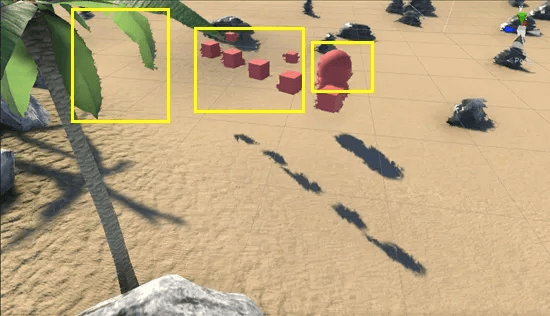3. WaterDepth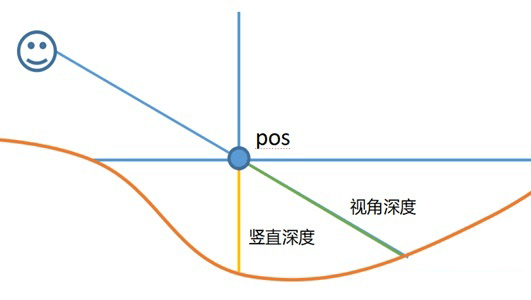WaterDepth示意图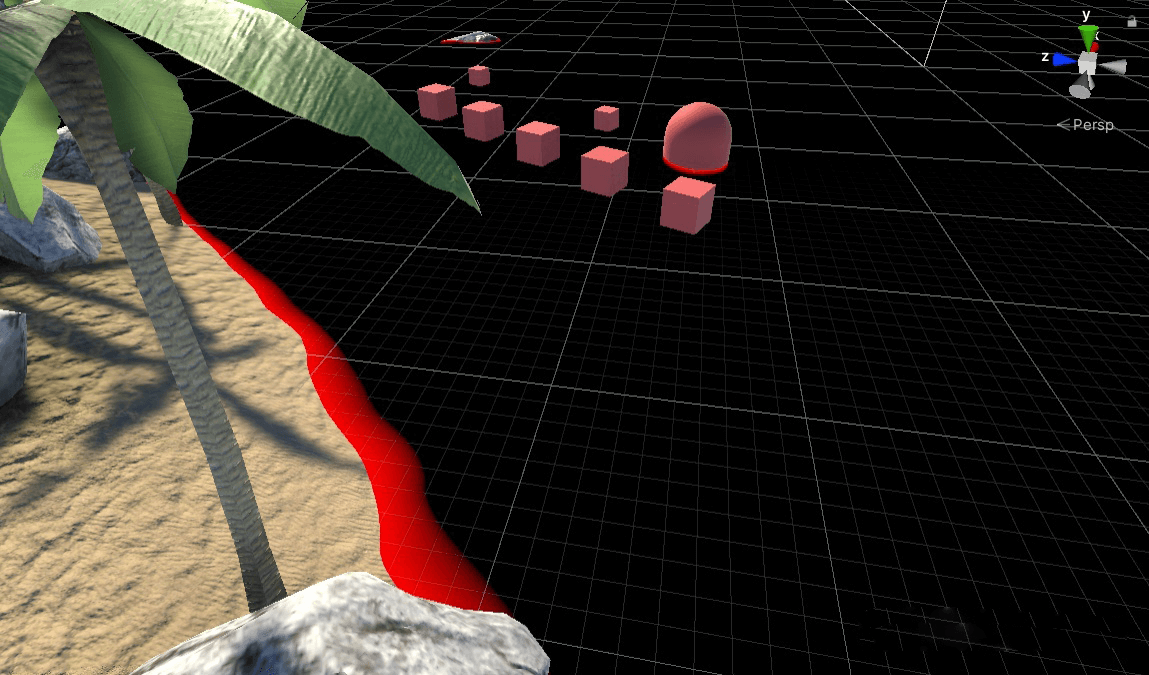WaterDepth.x

WaterDepth.y 添加一个垂直水平面的相机，生成深度纹理。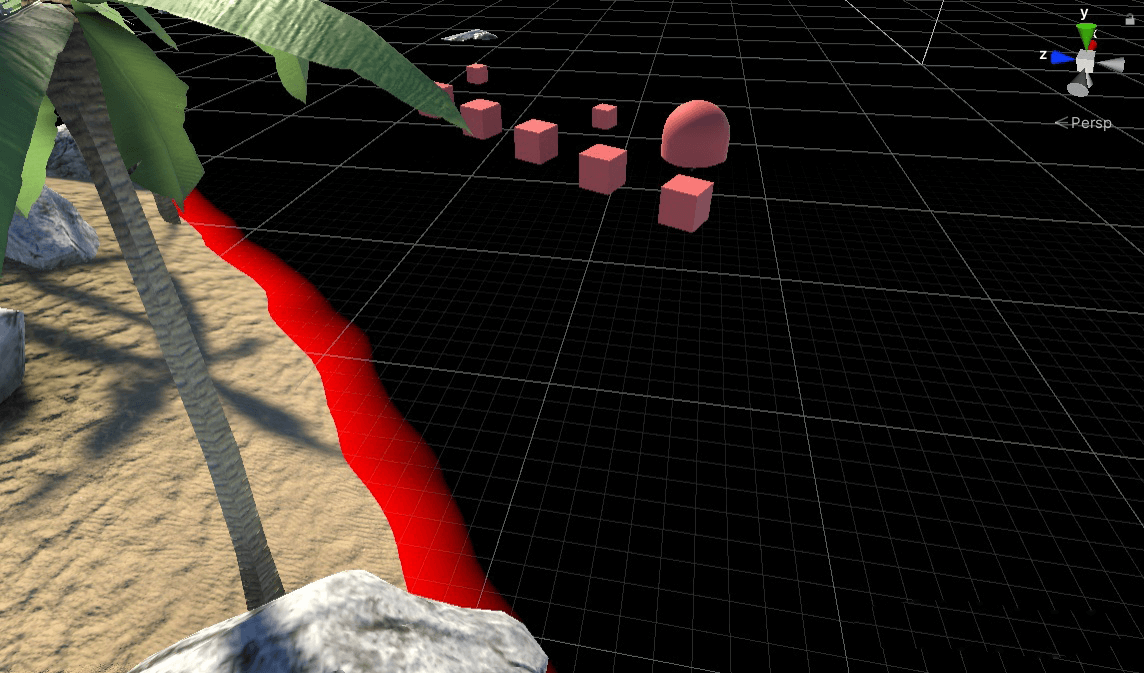WaterDepth.y

4. 修正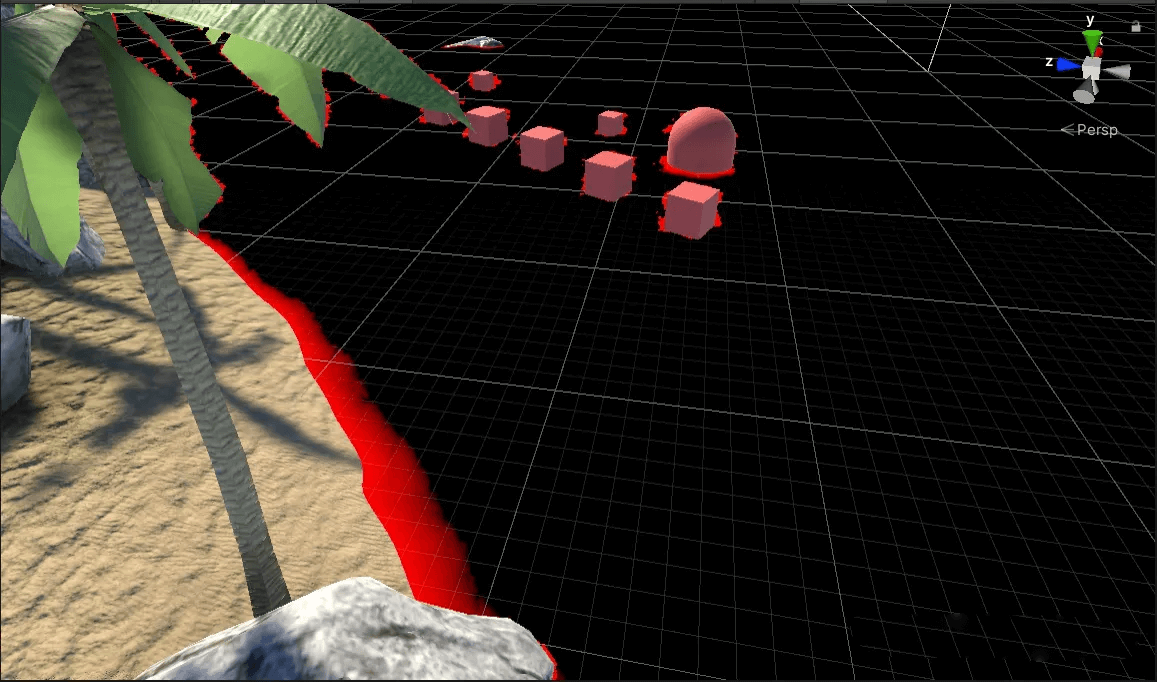WaterDepth.x（修正）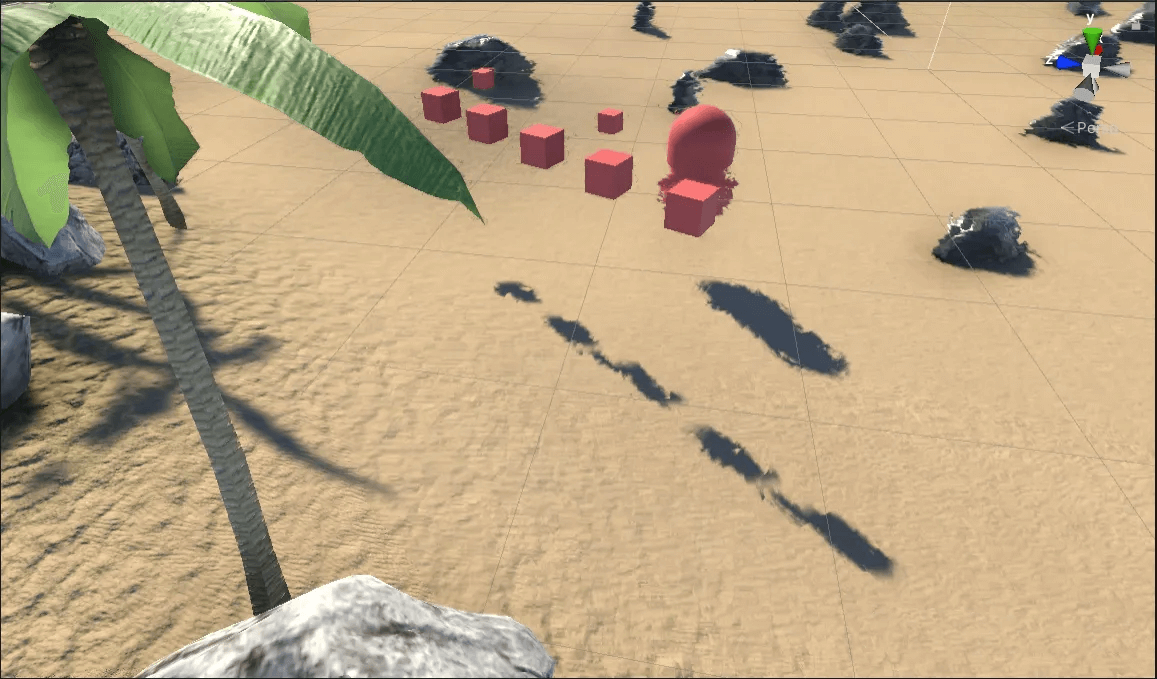5. SSS吸收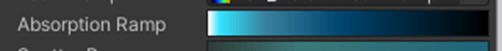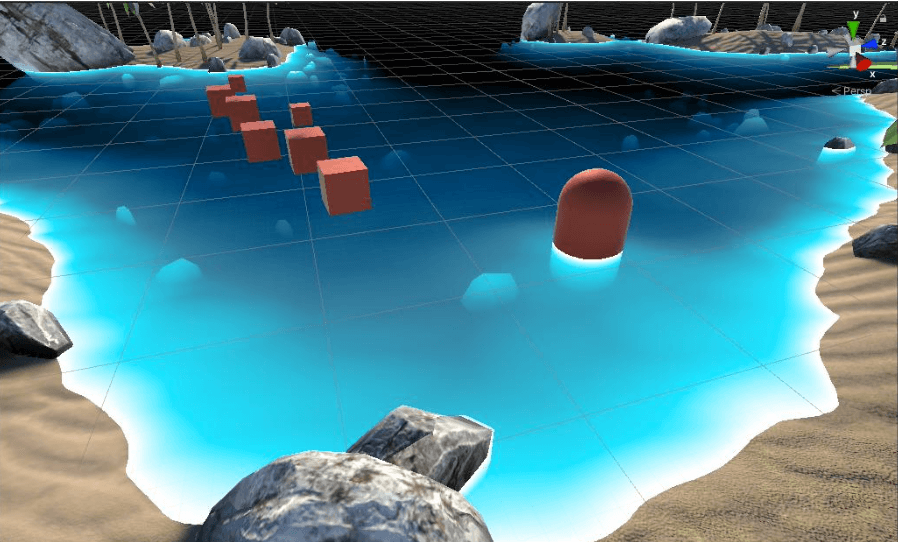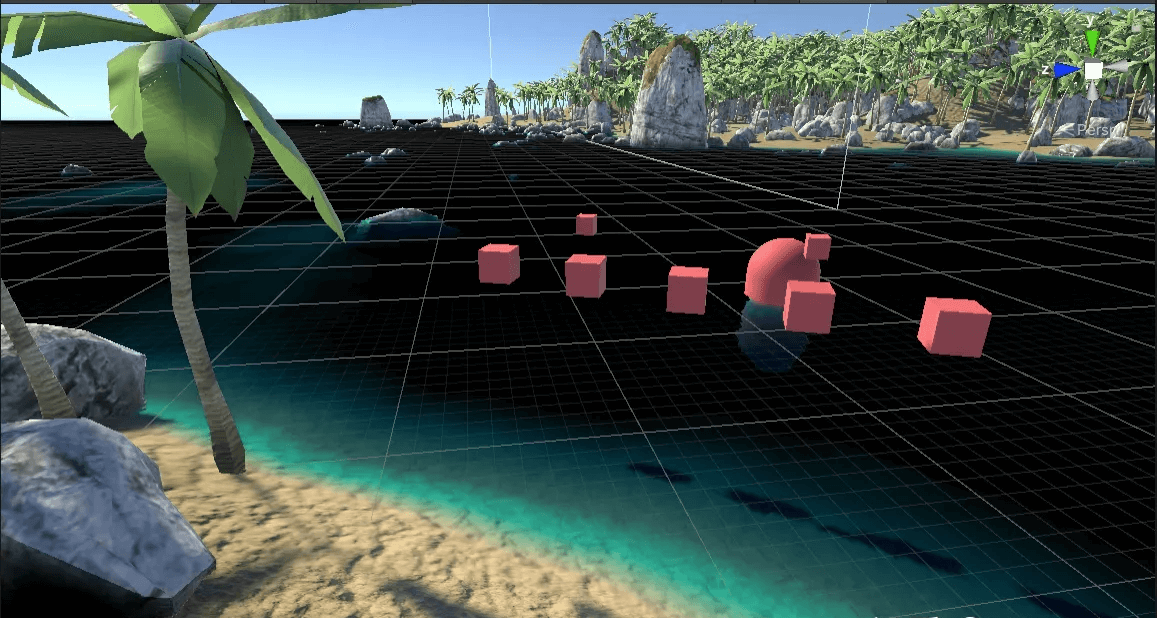### 三、反射

1. 翻转摄像机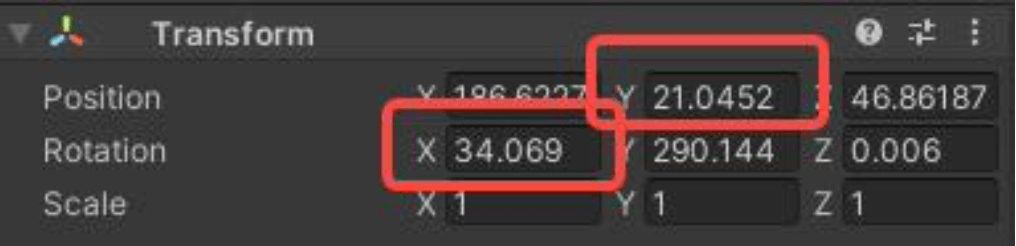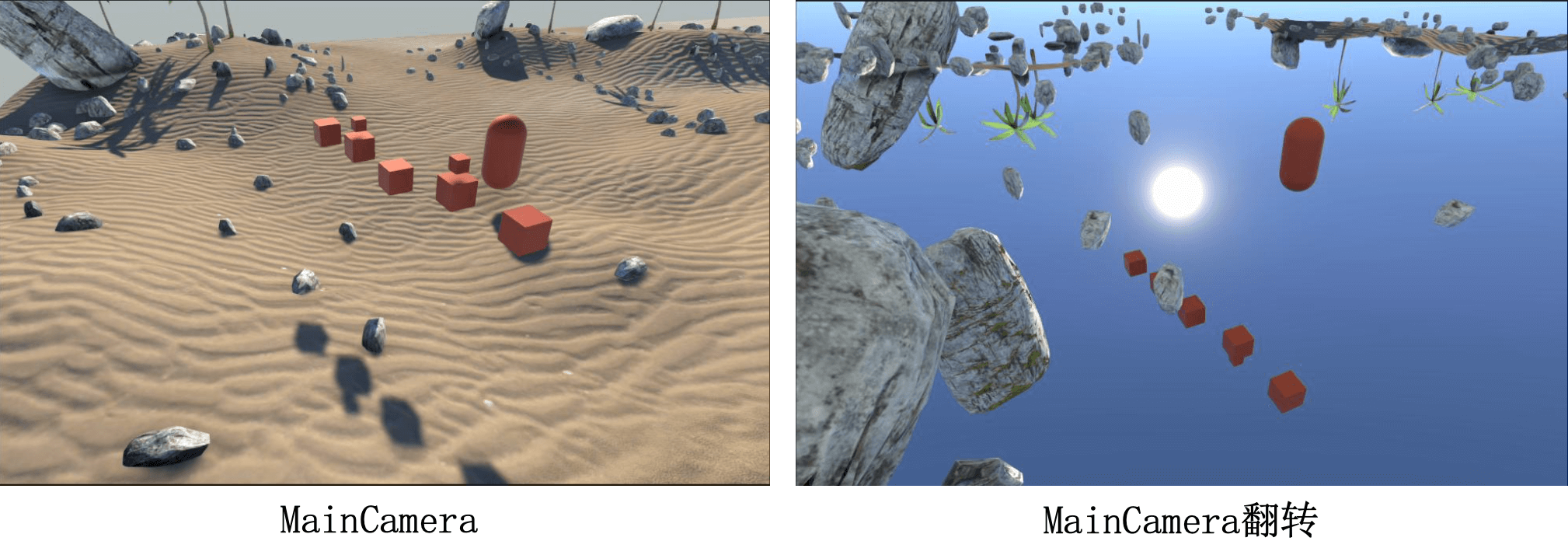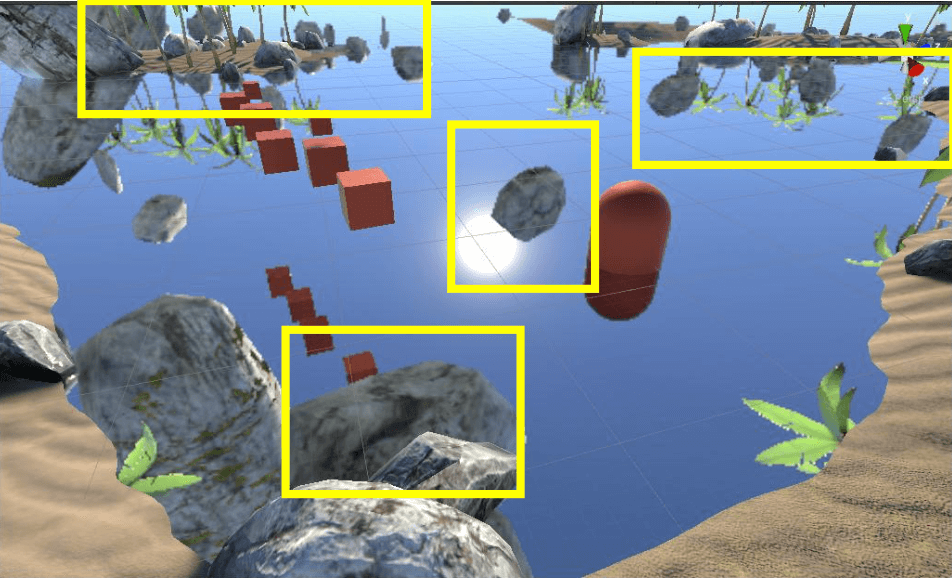2. 斜面裁剪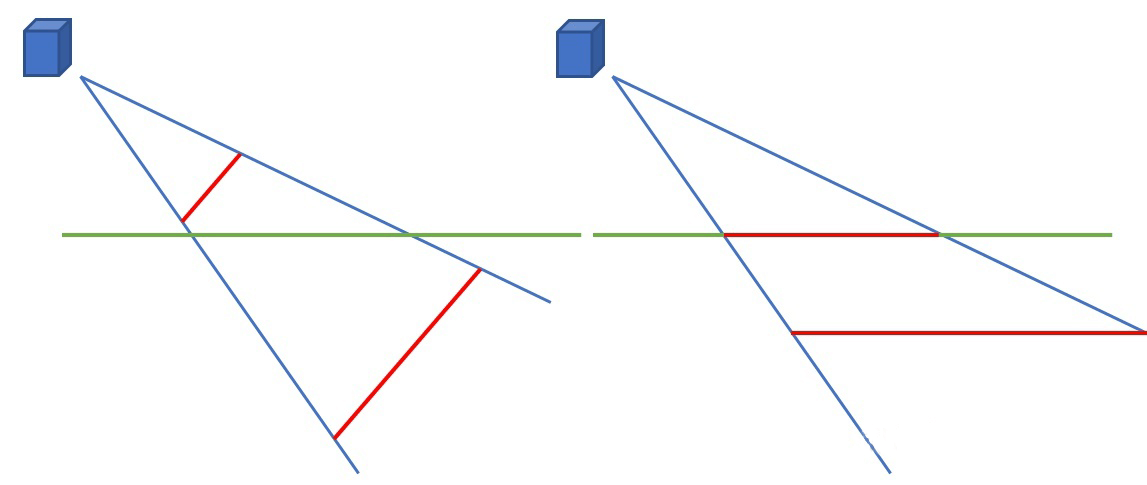Unity里可以很方便地实现斜面裁剪，调用CameraSpacePlane()，设置好裁剪面即可。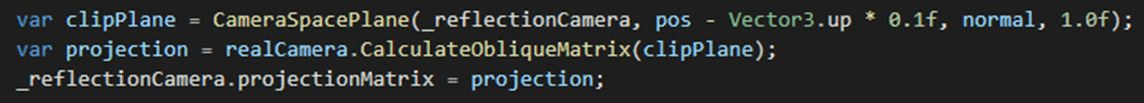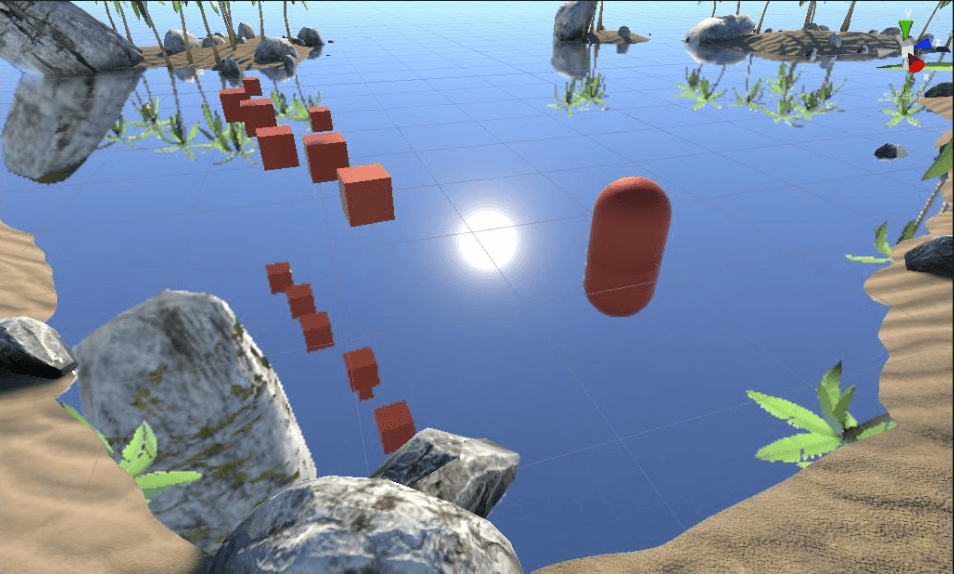3. UV扰动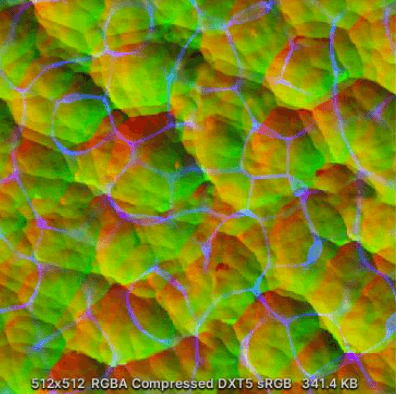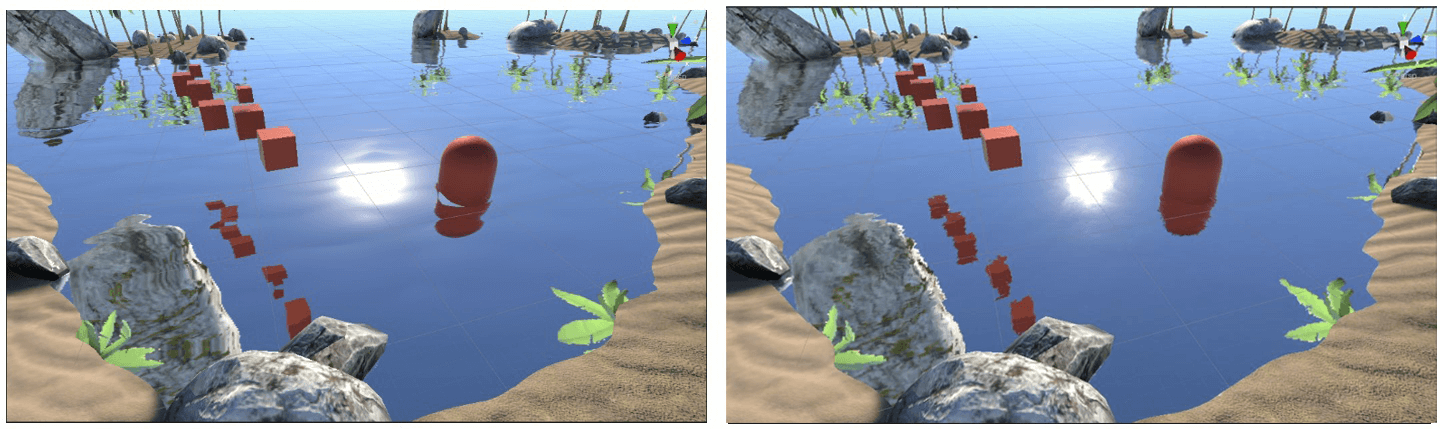### 四、菲涅尔项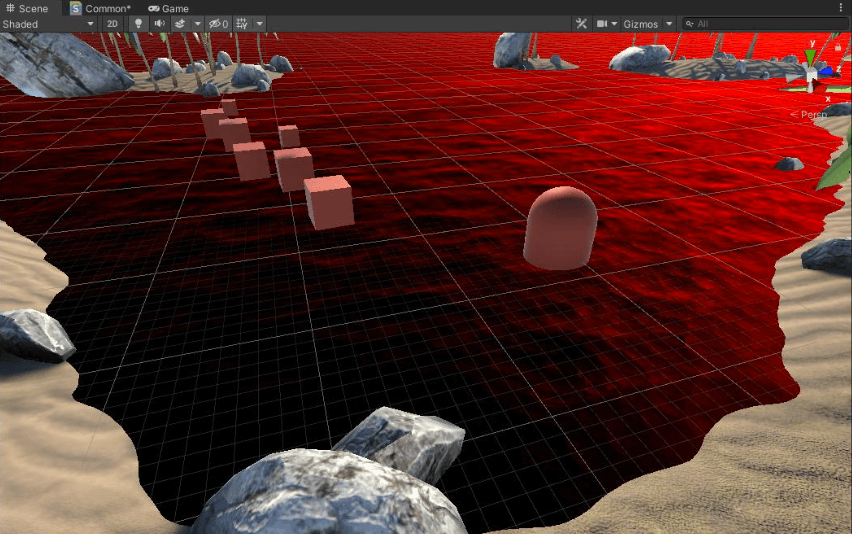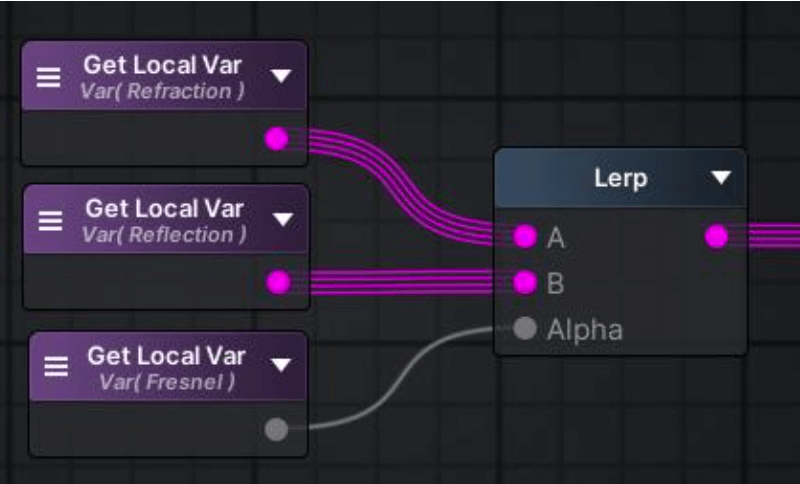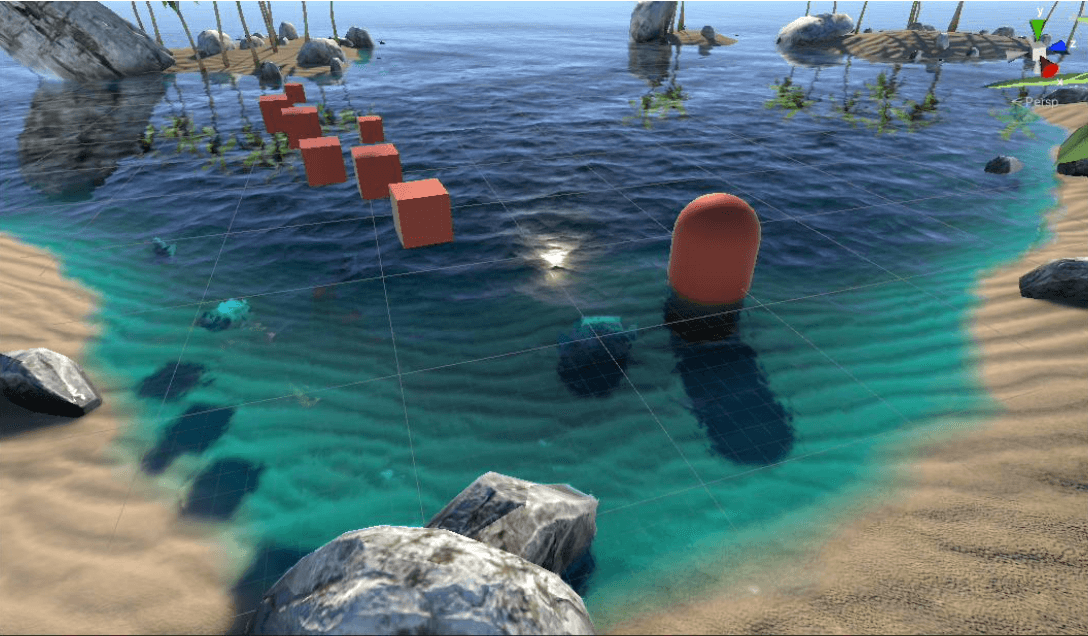### 五、SSS散射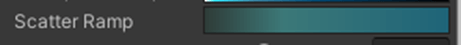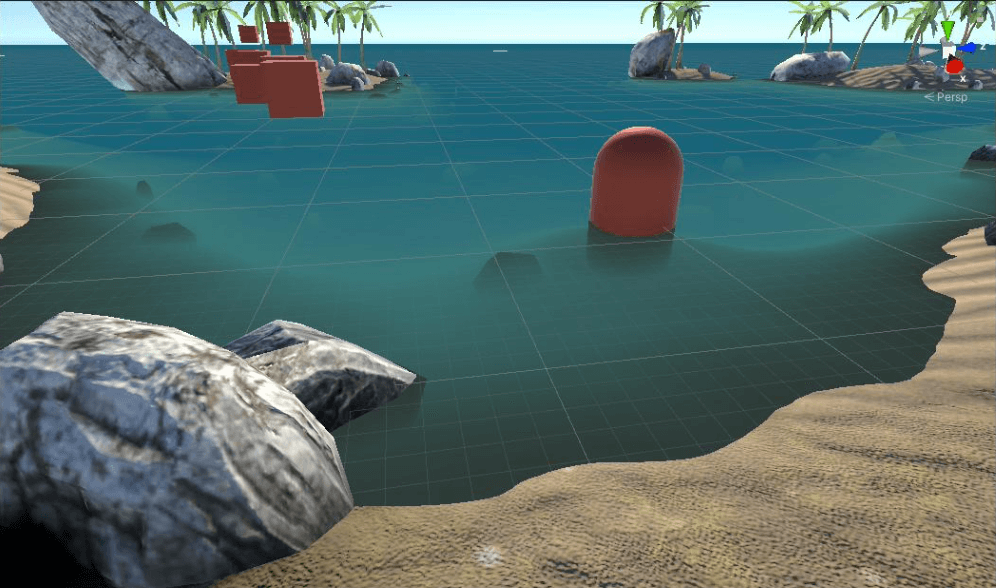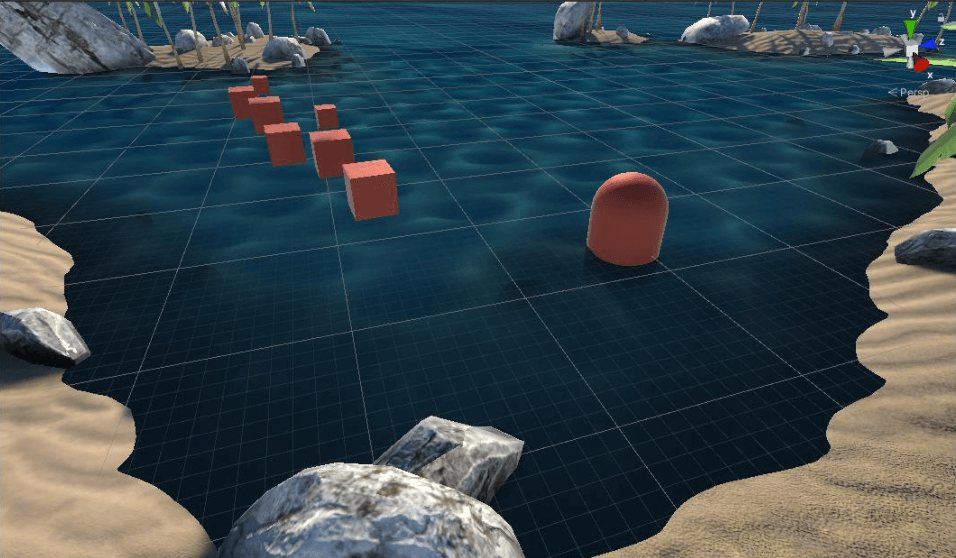SSS散射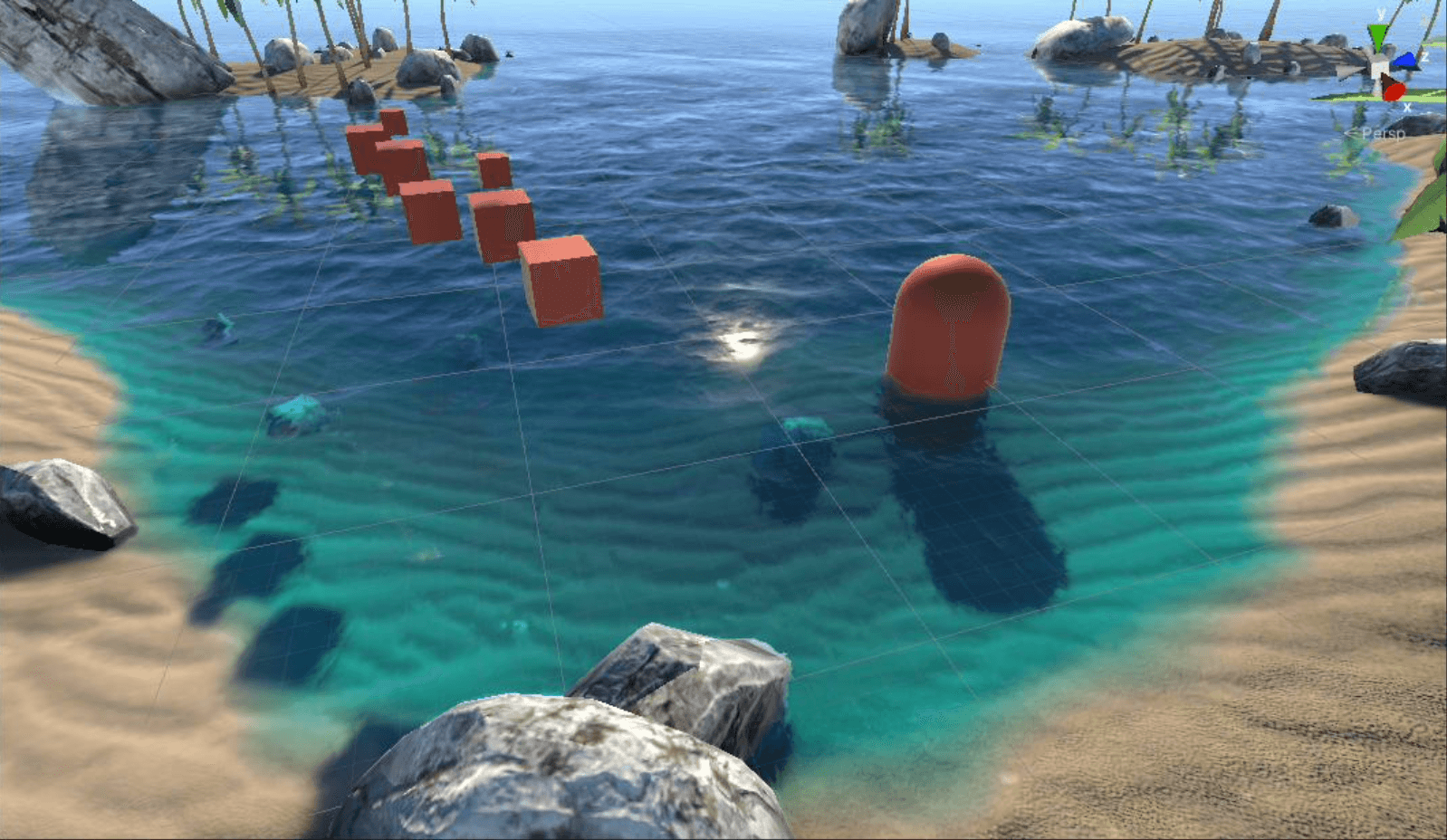### 六、高光反射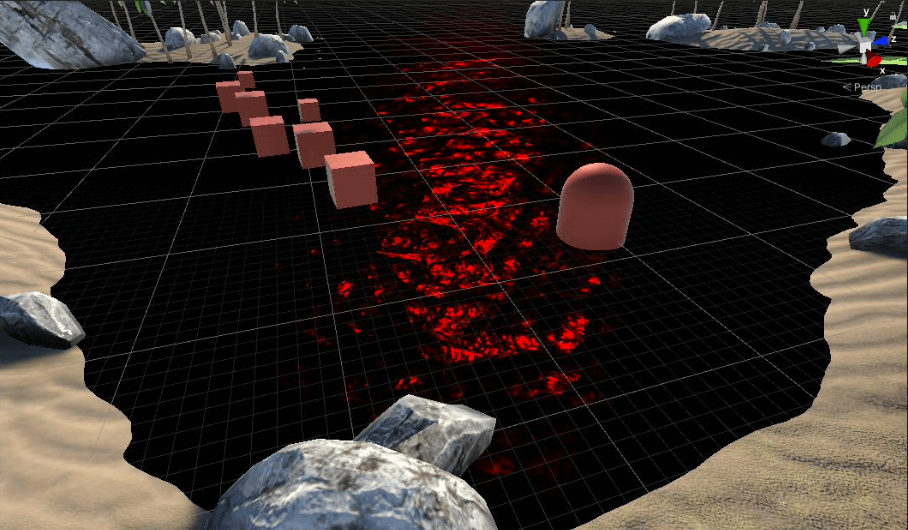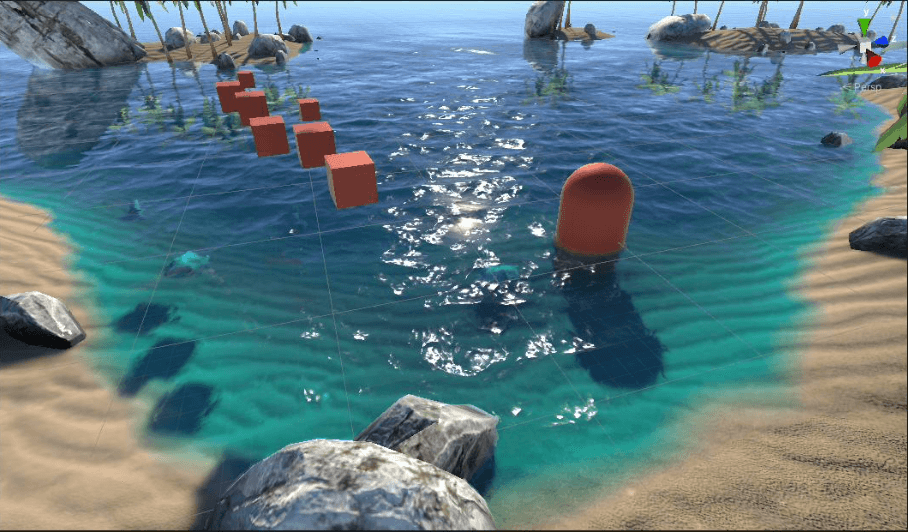### 七、浮沫

1. 深度采样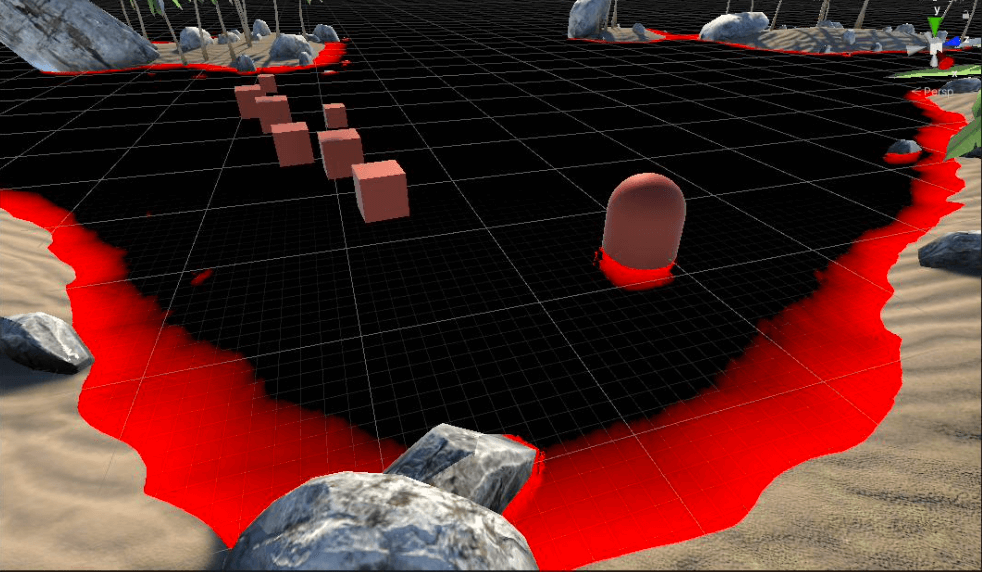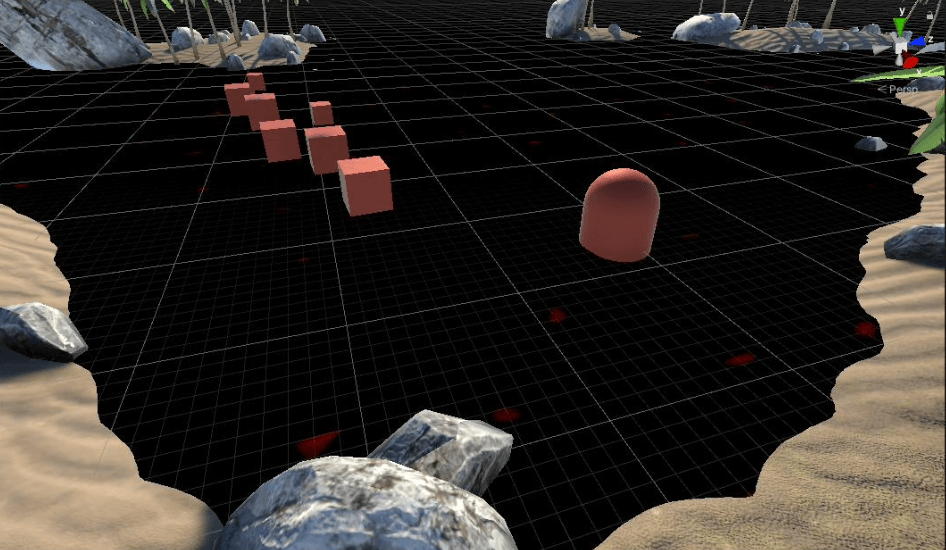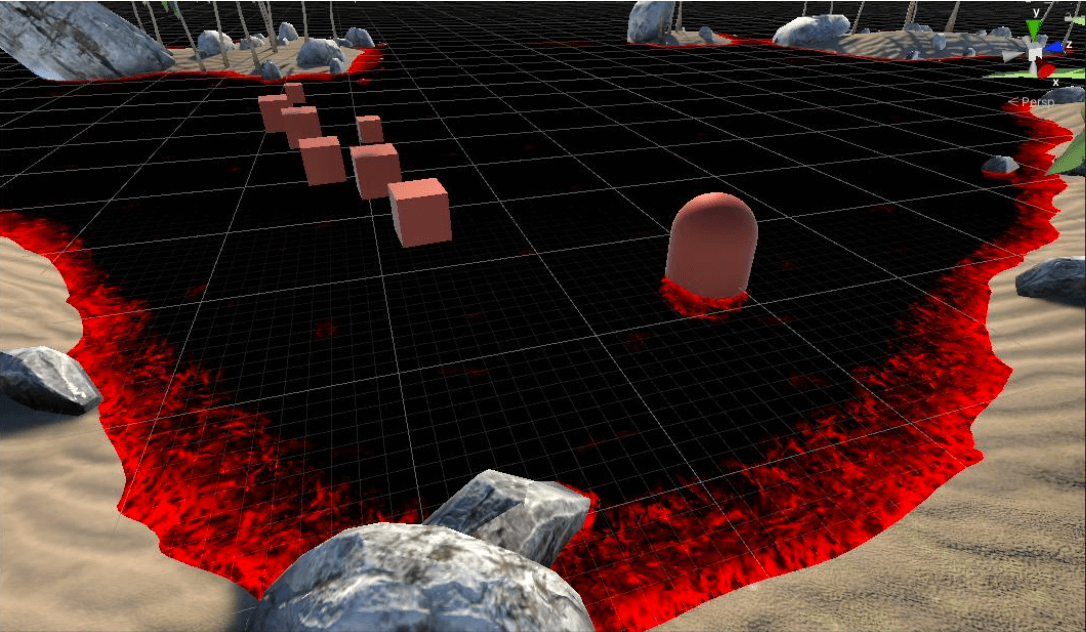2. 计算光照
Demo里的浮沫本身没有颜色，需要通过浮沫遮罩计算光照得到：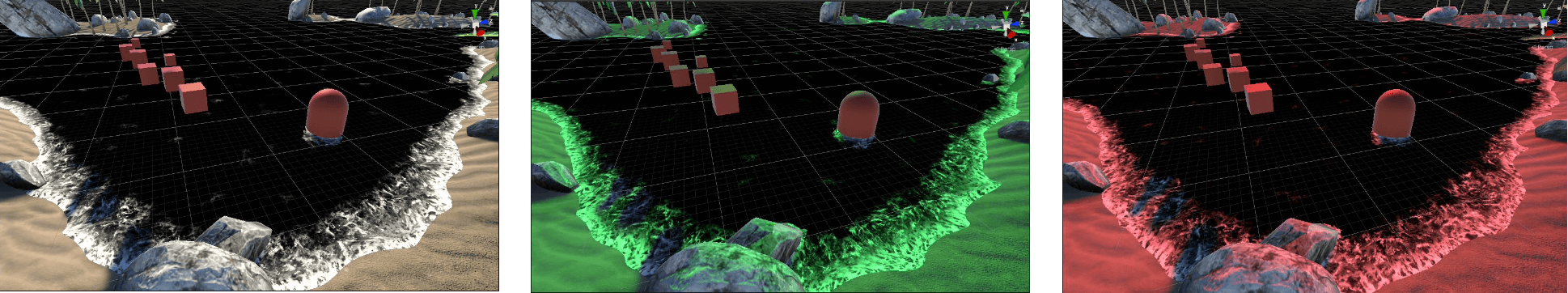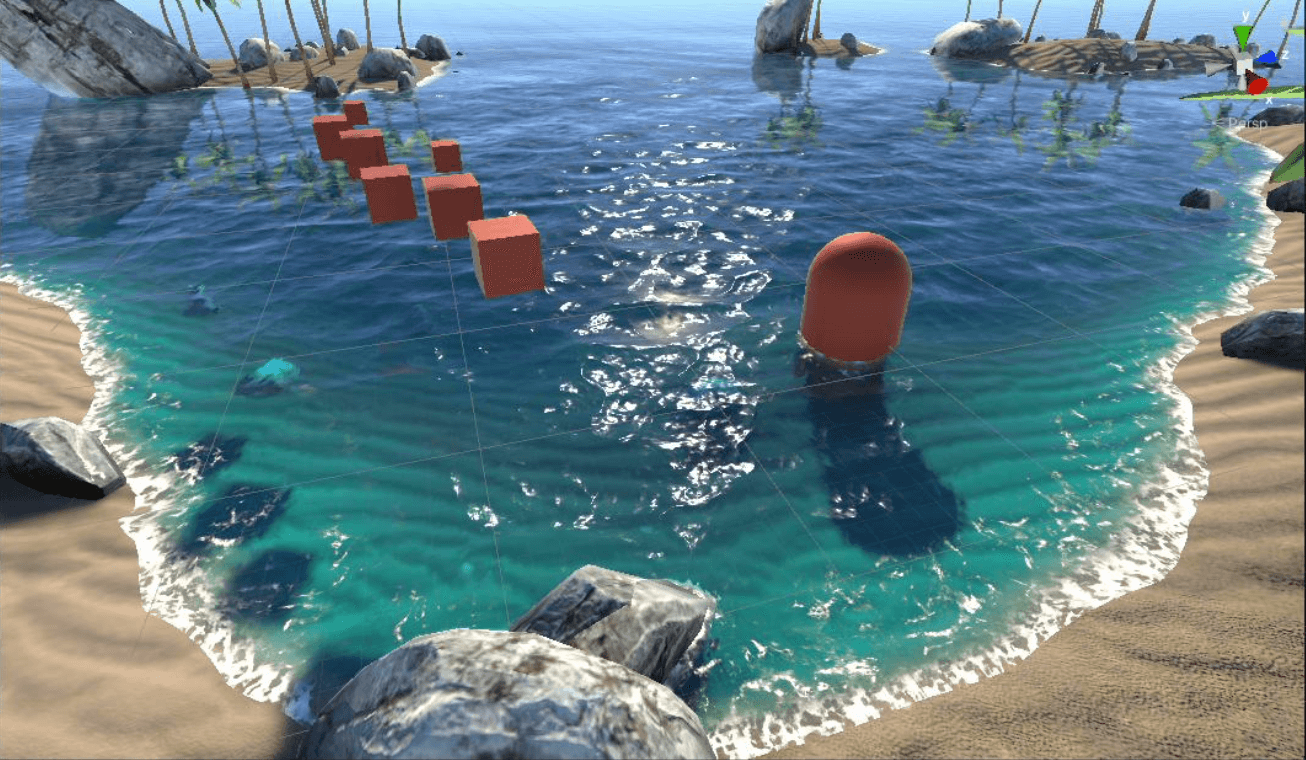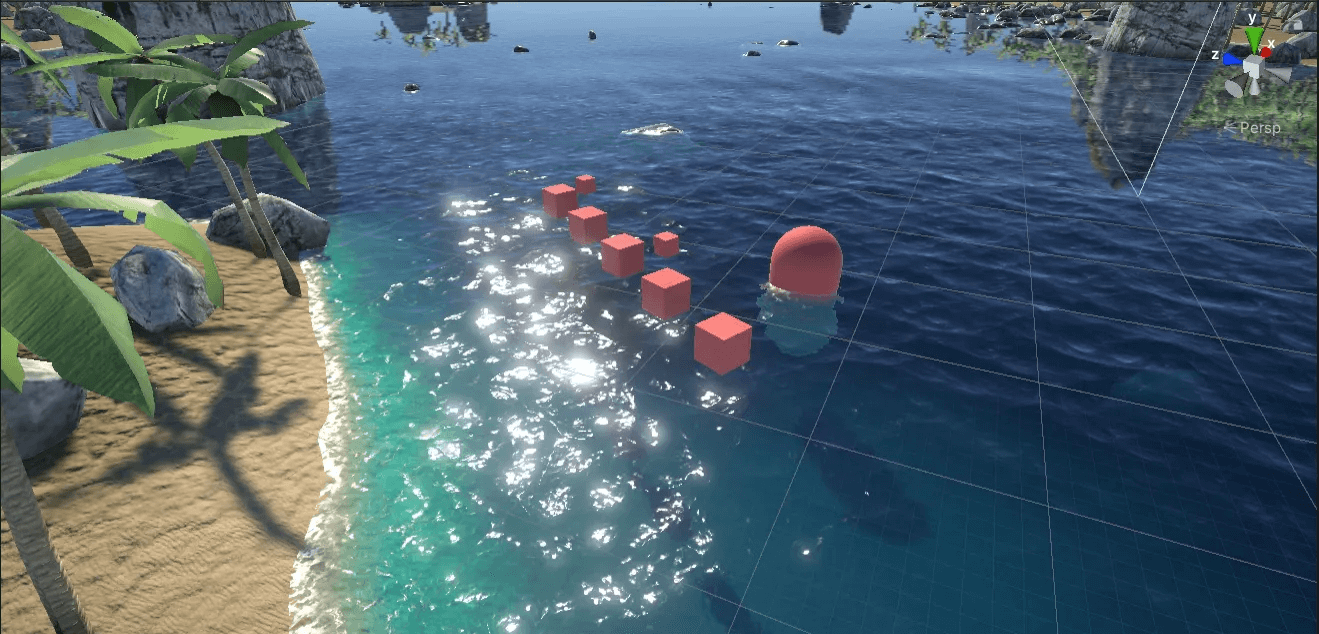Bloom后处理

lionheart：BoatAttack_水效果分析2_水的细节作色效果（折射，SSS，高光）

jaleco：Boat Attack 项目海水技术解析

https://github.com/Unity-Techno